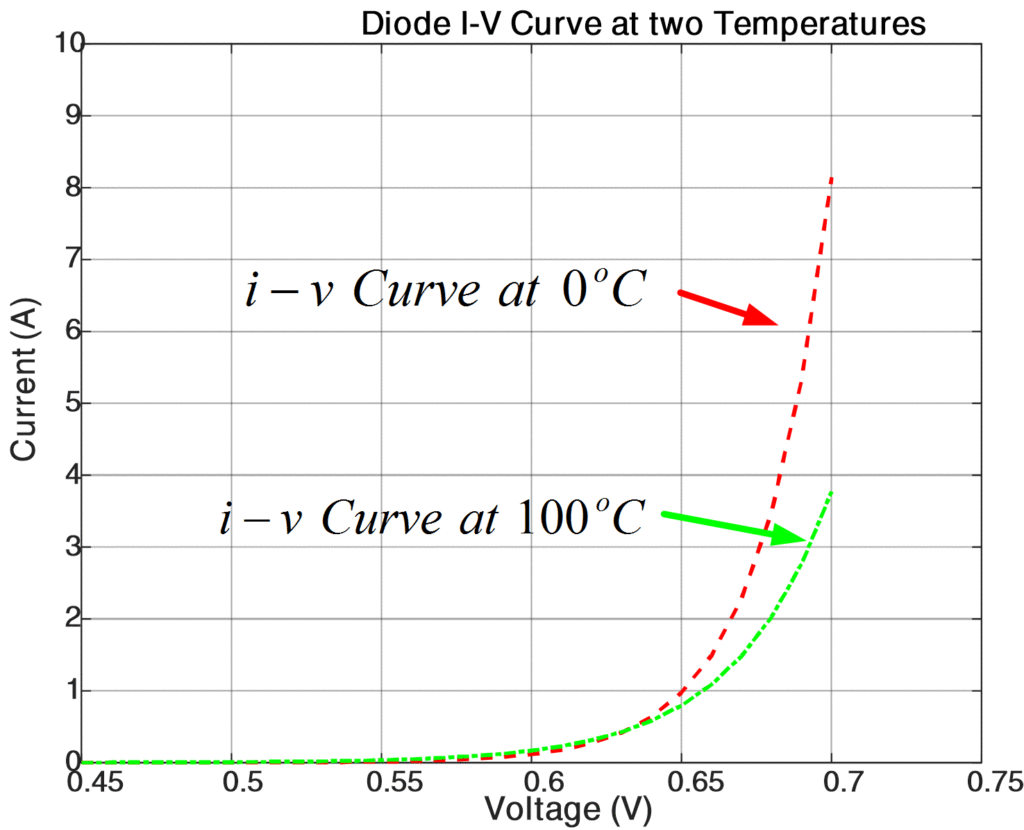Home / Circuits with Matlab / Diode Characteristic Curve Calculation at Different Temperatures using Matlab

# Diode Characteristic Curve Calculation at Different Temperatures using Matlab

Want create site? Find Free WordPress Themes and plugins.

In this article, we will draw characteristic curves of a diode at different temperatures.

From the following equation, it is evident that the thermal voltage and the reverse saturation current of a diode depend on the temperature.

$i={{I}_{s}}\left[ {{e}^{\left( {}^{v}/{}_{n{{V}_{T}}} \right)}}-1 \right]\text{ }\cdots \text{ (1)}$

IS is reverse saturation current or leakage current,

n is an empirical constant between 1 and 2,

VT is thermal voltage, given by

$\begin{matrix} {{V}_{T}}=\frac{kT}{q} & \cdots & (2) \\\end{matrix}$

k is Boltzmann’s constant = 1.38×10−23 J / oK,

q is the electronic charge = 1.6×10−19 Coulombs,

T is the absolute temperature in oK

At room temperature (25 oC), the thermal voltage is about 25.7 mV.

The reverse saturation current Is of a diode increases by 7.2%/0C for germanium as well as for silicon material. The reverse saturation current can be expressed as:

$\begin{matrix} {{I}_{s}}({{T}_{2}})={{I}_{s}}({{T}_{1}}){{e}^{\left[ {{k}_{s}}({{T}_{2}}-{{T}_{1}}) \right]}} & \cdots & (3) \\\end{matrix}$

Where

${{k}_{s}}=0.072/{}^{o}C$

And T1 and T2 represent different temperatures.

Example:

Saturation current of a diode at 25oC=10-12 A

Emission Constant=1.9

Let’s plot the v-i curves at the following temperatures: T1 = 0 0C, T2 = 100 0C using Matlab:

% Temperature effects on diode characteristics
clear all;close all;clc
%%Parameters and Formulation Part
k_Boltz = 1.38e-23; % Boltzman Constant
q_Electron = 1.6e-19; % q is the electron charge
T1 = 273 + 0; %Temperature Conversion to Kelvin Scale
T2 = 273 + 100; %Temperature Conversion to Kelvin Scale
l_saturation1 = 1.0e-12; % Saturation Current
k_saturation = 0.072; % Saturation Constant
l_saturation2 = l_saturation1*exp(k_saturation*(T2-T1)); % Saturation Current mentioned in (3) in text
V_diode = 0.45:0.01:0.7; % Voltage Sampling
l_T1 = l_saturation1*exp(q_Electron*V_diode/(k_Boltz*T1)); % Diode Current at T1
l_T2 = l_saturation2*exp(q_Electron*V_diode/(k_Boltz*T2)); % Diode Current at T2
%% Plotting the Results
plot(V_diode,l_T1,'r',V_diode,l_T2,'g')
axis([0.45,0.75,0,10])
title('Diode I-V Curve at two Temperatures')
xlabel('Voltage (V)')
ylabel('Current (A)')


Results:Fig.1: Diode Characteristic Curves

Did you find apk for android? You can find new Free Android Games and apps.